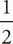# SAT Math Multiple Choice Question 64: Answer and Explanation

### Test Information

Question: 64

4. What is the equation of a line that contains the point (1, 6) and has a y-intercept of 4 ?

• A. y =x + 4
• B. y = x + 4
• C. y = 2x + 4
• D. y = 4x + 2

C All of the answers are written in the slope-intercept form y = mx + b, where b is the y-intercept and x and y are points on the line. Eliminate (D) because the y-intercept in that equation is 2. For the remaining answer choices, plug in the x- and y-values to determine which equationworks. If x = 1 and y = 6, (A) becomes 6 =(1) + 4. Solve both sides of the equation to get 6 = 4. Eliminate (A). Choice (B) becomes 6 = 1 + 4, so eliminate (B). Choice (C) becomes 6 = 2(1) + 4, or 6 = 6. Therefore, the correct answer is (C).